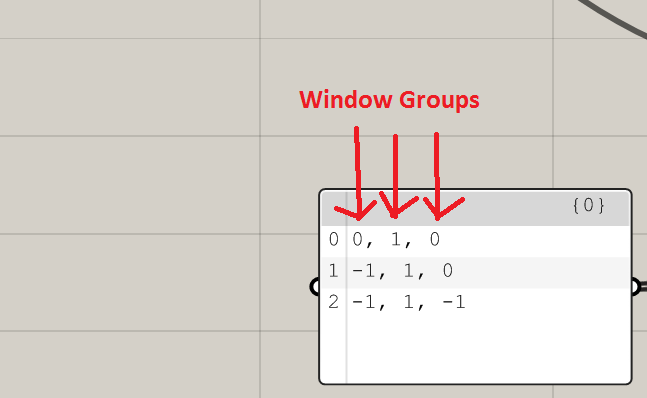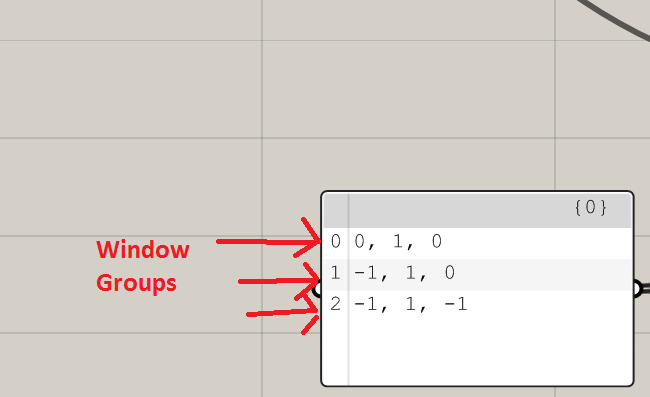# Trying to understand blindCombs window sequence

Hi Everyone,

I was going through the 3-phase example and was trying to understand the window group sequence used in the blindCombs input of the blind states component.

The image above shows 3 steps used on 3 window groups.

1. (0,1,0)
2. (-1,1,0)
3. (-1,1,-1)

From the description in the image above I interpret the window groups being assigned to
to the values like this.1. (0,1,0) = (side window, south window, skylight) = (clear, diffuse, clear).
2. (-1,1,0) = (side window, south window, skylight) = (closed, diffuse, clear).
3. (-1,1,-1) = (side window, south window, skylight) = (closed, diffuse, closed).
is that right?
or does the window group get applied to the index value like this:1. south window (0,1,0) = (clear, diffuse, clear)
2. side window (-1,1,0) = (closed, diffuse, clear)
3. skylight (-1,1,-1) = (closed, diffuse, closed)

1 Like

This one is correct. Each row is the combination of states for different window groups. It gives you a flexible solution to define dynamic blinds logic for each AnalysisGrid/Zone.

2 Likes

I’m working with 4 states for each windows groups. You write the blinds-state as following:
(0,0) = (generic window non blinds, window group with venetian blinds) = (clear, blind open).
(0,1) = (generic window non blinds, window group with venetian blinds) = (clear, blind closed 25° above orizzont).
(0,2) = (generic window non blinds, window group with venetian blinds) = (clear, blind closed 45°).
(0,3) = (generic window non blinds, window group with venetian blinds) = (clear, blind closed 60°).

… and works fine

2 Likes

Yes. This is correct. Just to add a little bit more details all the normal windows are grouped together as the first window group. See this post for more details: Three phase vs five phase component resulting batch file calculations

2 Likes